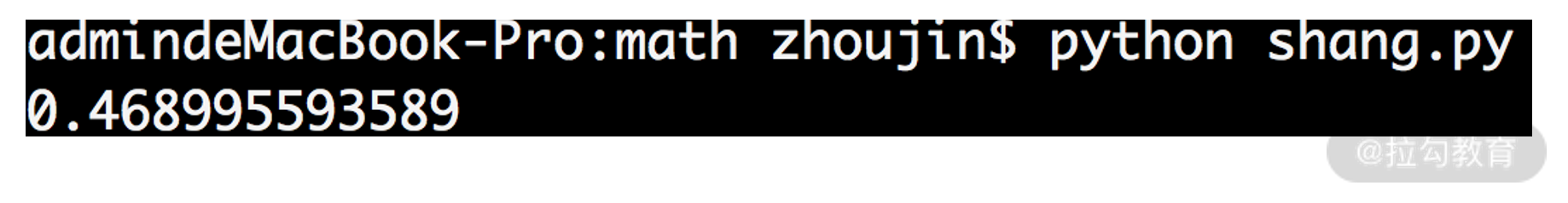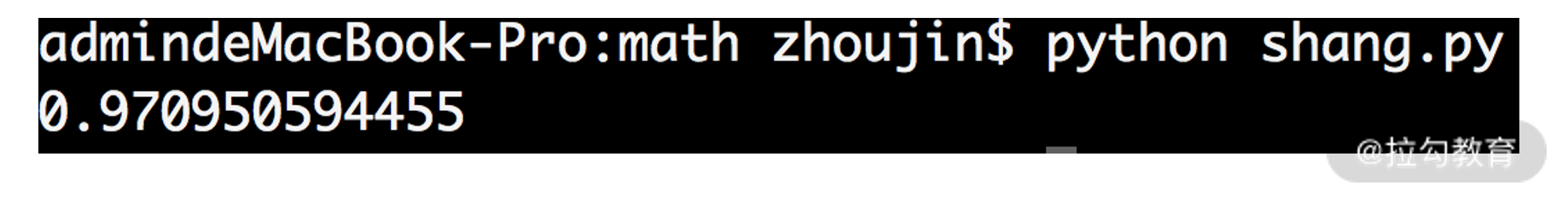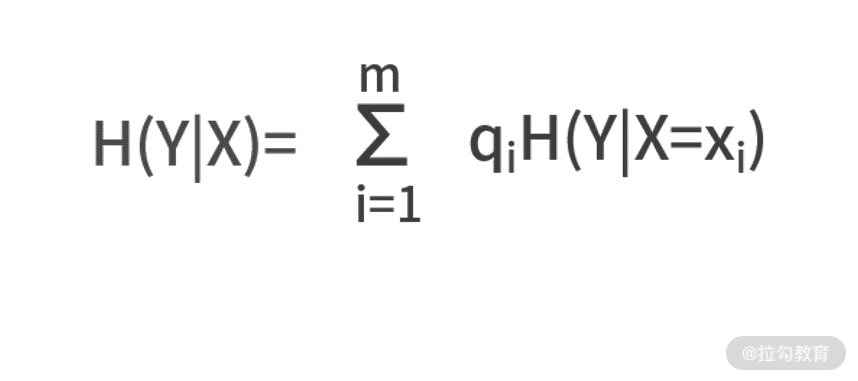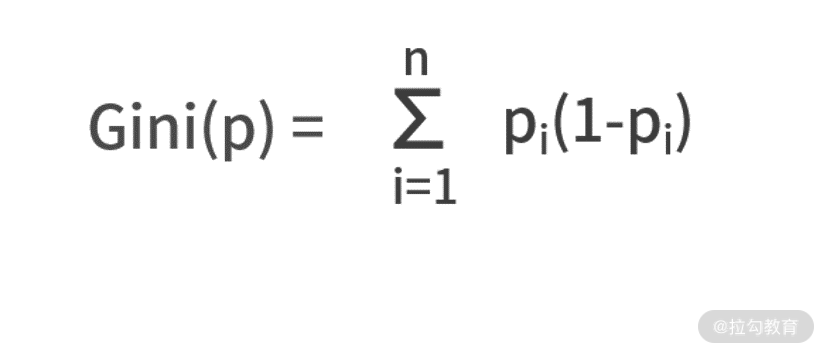10 信息熵：事件的不确定性如何计算？

熵import math

def entropy(*c):

result = 0

islegal = 0

for x in c:

islegal += x

result = result + (-x) * math.log(x,2)

if islegal != 1:

return 'input prob error!'

return result

if __name__ == '__main__':

print entropy(0.9,0.1)

• 从第 6 行开始，对输入的每个概率值进行循环。
• 每次循环的动作是，第 7 行计算概率的求和，并用 islegal 变量保存。
• 第 8 行，根据公式来计算熵的值，用 result 变量来保存。
• 最终，判断概率之和是否为 1，如果不是，则输出错误信息；如果没问题，则返回 result。条件熵• 健康的情况下，H(Y|X=x1) =-0.9×log20.9-0.1×log20.1 = 0.4690；
• 患病的情况下，H(Y|X=x2) =-0.6×log20.6-0.4×log20.4 = 0.9710。

信息增益可以描述条件熵和熵的关系

“熵”的含义是不确定性，而“条件熵”的含义是知道了某个条件下的不确定性。因此直觉来看，条件熵应该小于或等于熵，因为增加了“某个条件”就等于是知道了某个信息，最不济就是个无用信息，但无论如何一定会让“不确定性”减小。

• 信息增益，顾名思义就是信息量增加了多少；换句话说，也是不确定性降低了多少。标记为 g(X,Y)，定义式为 g(X,Y) = H(Y) - H(Y|X)。
• 有时候，除了看这个差值以外，还会同时观察降幅的比值。此时为信息增益率，定义式为 gr(X,Y) = g(X,Y) / H(Y)。

基尼系数• 对于巴西队的比赛而言，其基尼系数为 Gini(p) = 0.9*(1-0.9) + 0.1*(1-0.1) = 0.18
• 对于阿根廷对的比赛而言，其基尼系数为 Gini(p) = 0.4*(1-0.4) + 0.6*(1-0.6) = 0.48

小结

• 概率，描述的是某个事件的结果，发生的可能性。有时候，在不刻意强调区分“事件”和“事件结果”的时候，也被简称为事件发生的可能性。
• ，描述的则是事件背后蕴含的信息量和不确定性。